International
Tables for
Crystallography
Volume C
Mathematical, physical and chemical tables
Edited by E. Prince

International Tables for Crystallography (2006). Vol. C, ch. 4.3, pp. 414-415

Section 4.3.6.1. The multislice method

D. F. Lynchh

4.3.6.1. The multislice method

| top | pdf |

The calculation of very large numbers of diffracted orders, i.e. more than 100 and often several thousand, requires the multislice procedure. This occurs because, for N diffracted orders, the multislice procedure involves the manipulation of arrays of size N, whereas the scattering matrix or the eigenvalue procedures involve manipulation of arrays of size N by N.

The simplest form of the multislice procedure presumes that the specimen is a parallel-sided plate. The surface normal is usually taken to be the z axis and the crystal structure axes are often chosen or transformed such that the c axis is parallel to z and the a and b axes are in the xy plane. This can often lead to rather unconventional choices for the unit-cell parameters. The maximum tilt of the incident beam from the surface normal is restricted to be of the order of 0.1 rad. For the calculation of wave amplitudes for larger tilts, the structure must be reprojected down an axis close to the incident-beam direction. For simple calculations, other crystal shapes are generally treated by the column approximation, that is the crystal is presumed to consist of columns parallel to the z axis, each column of different height and tilt in order to approximate the desired shape and variation of orientation.

The numerical procedure involves calculation of the transmission function through a thin slice, calculation of the vacuum propagation between centres of neighbouring slices, followed by evaluation in a computer of the iterated equation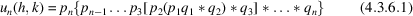in order to obtain the scattered wavefunction,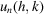, emitted from slice n, i.e. for crystal thickness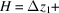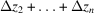; the symbol * indicates the operation convolution' defined by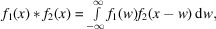and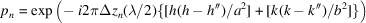is the propagation function in the small-angle approximation between slice n − 1 and slice n over the slice spacing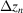. For simplicity, the equation is given for orthogonal axes and h′′, k′′ are the usually non-integral intercepts of the Laue circle on the reciprocal-space axes in units of (1/a), (1/b). The excitation errors,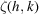, can be evaluated using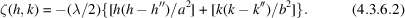The transmission function for slice n is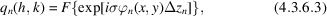where F denotes Fourier transformation from real to reciprocal space, and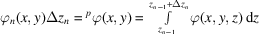and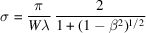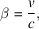where W is the beam voltage, v is the relativistic velocity of the electron, c is the velocity of light, and λ is the relativistic wavelength of the electron.

The operation * in (4.3.6.1)is most effectively carried out for large N by the use of the convolution theorem of Fourier transformations. This efficiency presumes that there is available an efficient fast-Fourier-transform subroutine that is suitable for crystallographic computing, that is, that contains the usual crystallographic normalization factors and that can deal with a range of values for h, k that go from negative to positive. Then,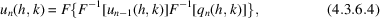where F denotes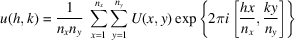and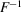denotes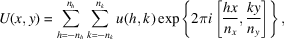where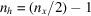,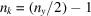, and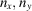are the sampling intervals in the unit cell. The array sizes used in the calculations of the Fourier transforms are commonly powers of 2 as is required by many fast Fourier subroutines. The array foris usually defined over the central portion of the reserved computer array space in order to avoid oscillation in the Fourier transforms (Gibbs instability). It is usual to carry out abeam calculation in an array of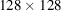, hence the critical timing interval in a multislice calculation is that interval taken by a fast Fourier transform for 4N coefficients. If the number of beams, N, is such that there is still appreciable intensity being scattered outside the calculation aperture, then it is usually necessary to impose a circular aperture on the calculation in order to prevent the symmetry of the calculation aperture imposing itself on the calculated wavefunction. This is most conveniently achieved by setting all p(h, k) coefficients outside the desired circular aperture to zero.

It is clear that the iterative procedure of (4.3.6.1)means that care must be taken to avoid accumulation of error due to the precision of representation of numbers in the computer that is to be used. Practical experience indicates that a precision of nine significant figures (decimal) is more than adequate for most calculations. A precision of six to seven (decimal) figures (a common 32-bit floating-point representation) is only barely satisfactory. A computer that uses one of the common 64-bit representations (12 to 16 significant figures) is satisfactory even for the largest calculations currently contemplated.

The choice of slice thickness depends upon the maximum value of the projected potential within a slice and upon the validity of separation of the calculation into transmission function and propagation function. The second criterion is not severe and in practice sets an upper limit to slice thickness of about 10 Å. The first criterion depends upon the atomic number of atoms in the trial structure. In practice, the slice thickness will be too large if two atoms of medium to heavy atomic weight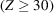are projected onto one another. It is not necessary to take slices less than one atomic diameter for calculations for fast electron (acceleration voltages greater than 50 keV) diffraction or microscopy. If the trial structure is such that the symmetry of the diffraction pattern is not strongly dependent upon the structure of the crystal parallel to the slice normal, then the slices may be all identical and there is no requirement to have a slice thickness related to the periodicity of the structure parallel to the surface normal. This is called the no upper-layer-line' approximation. If the upper-layer lines are important, then the slice thickness will need to be a discrete fraction of the c axis, and the contents of each slice will need to reflect the actual atomic contents of each slice. Hence, if there were four slices per unit cell, then there would need to be four distinct q(h, k), each taken in the appropriate order as the multislice operation proceeds in thickness.

The multislice procedure has two checks that can be readily performed during a calculation. The first is applied to the transmission function, q(h, k), and involves the evaluation of a unitarity test by calculation of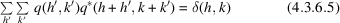for all h, k, where q* denotes the complex conjugate of q, and δ(h,k) is the Kronecker delta function. The second test can be applied to any calculation for which no phenomenological absorption potential has been used in the evaluation of the q(h, k). In that case, the sum of intensities of all beams at the final thickness should be no less than 0.9, the incident intensity being taken as 1.0. A value of this sum that is less than 0.9 indicates that the number of beams, N, has been insufficient. In some rare cases, the sum can be greater than 1.0; this is usually an indication that the number of beams has been allowed to come very close to the array size used in the convolution procedure. This last result does not occur if the convolution is carried out directly rather than by use of fast-Fourier-transform methods.

A more complete discussion of the multislice procedure can be obtained from Cowley (1975) and Goodman & Moodie (1974). These references are not exhaustive, but rather an indication of particularly useful articles for the novice in this subject.

References

Cowley, J. M. (1975). Diffraction physics. New York: North-Holland.
Goodman, P. & Moodie, A. F. (1974). Numerical evaluation of N-beam wave-functions in electron scattering by the multislice method. Acta Cryst. A30, 280–290.